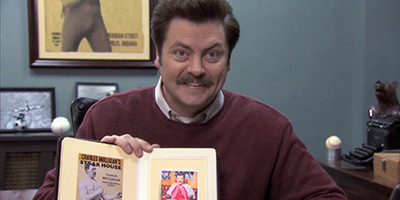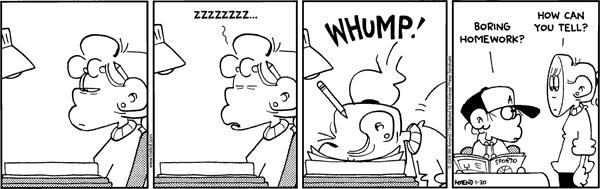## Exercise 1

Before it closed, Ron Swanson was a frequent patron of Charles Mulligan’s Steakhouse in Indianapolis, Indiana. Ron enjoyed the experience so much, during each visit he took a picture with his steak.Ron also weighed each steak he consumed. He has a record of eating six “22 ounce” Charles Mulligan’s porterhouse steaks. Ron found that these six steaks weighed

$22.4 \text{ oz}, \ 20.8 \text{ oz}, \ 21.6 \text{ oz}, \ 20.2 \text{ oz}, \ 21.4 \text{ oz}, \ 22.0 \text{ oz}$

Suppose that “22 ounce” Charles Mulligan’s porterhouse steaks follow a $$N(\mu, \sigma^2)$$ distribution and that Ron’s six steaks were a random sample.

(a) Compute the sample standard deviation, $$s$$, of these six steaks. Do not use a computer. You may only use $$+$$, $$-$$, $$\times$$, $$\div$$, and $$\sqrt{}$$ on a calculator. Show all work.

Solution:

$$x_i$$ $$x_i - \bar{x}$$ $$(x_i - \bar{x})^2$$
22.4 1.0 1.00
20.8 -0.6 0.36
21.6 0.2 0.04
20.2 -1.2 1.44
21.4 0 0.00
22.0 0.6 0.36

So, we have

$\sum_{i = n}^{n} x_i = 128.4$

Thus

$\bar{x} = \frac{1}{n} \sum_{i = n}^{n} x_i = \frac{1}{6}(128.4) = 21.4$

Note that,

$\sum_{i = n}^{n} (x_i - \bar{x}) = 0.$

More importantly,

$\sum_{i = n}^{n} (x_i - \bar{x})^2 = 3.2$

Thus,

$s^2 = \frac{1}{n - 1}\sum_{i = n}^{n} (x_i - \bar{x})^2 = \frac{1}{5}(3.2) = 0.64$

Then finally,

$s = \sqrt{s^2}= \sqrt{0.64} = \boxed{0.8}$

x = c(22.4, 20.8, 21.6, 20.2, 21.4, 22.0)
mean(x)
##  21.4
var(x)
##  0.64
sd(x)
##  0.8

(b) Construct a $$95\%$$ two-sided confidence interval for the true mean weight of a “22 ounce” Charles Mulligan’s porterhouse steak, $$\mu$$.

Solution:

Here $$\sigma$$ is unknown and $$n$$ is small, so we use $$t$$.

$\bar{x} \pm t_{\alpha / 2}(n - 1)\frac{s}{\sqrt{n}}$

We have,

• $$n = 6$$,
• $$\bar{x} = 21.4$$,
• $$s = 0.8$$,
• $$1 - \alpha = 0.95$$, so $$\alpha / 2 = 0.025$$,
• $$t_{\alpha / 2}(n - 1) = t_{0.025}(5) = 2.571$$.

$21.4 \pm 2.571 \frac{0.8}{\sqrt{6}}$

$\boxed{\bf 21.4 \pm 0.8397}$

$\boxed{\bf (20.5603, 22.2397)}$

n = length(x)
est = mean(x)
s = sd(x)
alpha = 0.05
crit = qt(alpha / 2, df = n - 1, lower.tail = FALSE)
se = s / sqrt(n)
margin = crit * se
lower = est - margin
upper = est + margin
c(lower, upper) # above answer contains some rounding, hence the difference
##  20.56045 22.23955
t.test(x, level = 0.95)\$conf.int
##  20.56045 22.23955
## attr(,"conf.level")
##  0.95

(c) Construct a $$95\%$$ confidence lower bound for the true mean weight of a “22 ounce” Charles Mulligan’s porterhouse steak, $$\mu$$.

Solution:

$\left[ \bar{x} - t_{\alpha}(n - 1)\frac{s}{\sqrt{n}}, \infty \right)$

We have,

• $$n = 6$$,
• $$\bar{x} = 21.4$$,
• $$s = 0.8$$,
• $$1 - \alpha = 0.95$$, so $$\alpha = 0.05$$,
• $$t_{\alpha}(n - 1) = t_{0.05}(5) = 2.0150$$.

$21.4 - 2.0150 \frac{0.8}{\sqrt{6}} = 20.7419$

$\boxed{[20.7419, \infty)}$

n = length(x)
est = mean(x)
s = sd(x)
alpha = 0.05
crit = qt(alpha, df = n - 1, lower.tail = FALSE)
se = s / sqrt(n)
margin = crit * se
(lower = est - margin)
##  20.74189

(d) Construct a $$90\%$$ two-sided confidence interval for the true standard deviation of the weight of a “22 ounce” Charles Mulligan’s porterhouse steak, $$\sigma$$.

Solution:

$\left(\sqrt{\frac{(n - 1) \cdot s^2}{\chi_{\alpha / 2}^2(n-1)}}, \sqrt{\frac{(n - 1) \cdot s^2}{\chi_{1 - \alpha / 2}^2(n-1)}} \right)$

• $$n = 6$$,
• $$s = 0.8$$,
• $$1 - \alpha = 0.90$$, so $$\alpha/2 = 0.05$$, and $$1 - \alpha/2 = 0.95$$
• $$\chi_{\alpha / 2}^2(n - 1) = \chi_{0.05}^2(5) = 11.07$$.
• $$\chi_{1 - \alpha / 2}^2(n-1) = \chi_{0.95}^2(5) = 1.145$$.

$\left( \sqrt{\frac{(6 - 1) \cdot 0.8^2}{11.07}} , \sqrt{\frac{(6 - 1) \cdot 0.8^2}{1.145}} \right)$

$\boxed{(0.5377, 1.6718)}$

n = 6
s = 0.8
alpha = 0.10
crit_lower = qchisq(alpha / 2, df = n - 1, lower.tail = FALSE)
crit_upper = qchisq(1 - alpha / 2, df = n - 1, lower.tail = FALSE)
lower = sqrt((n - 1) * s ^ 2 / crit_lower)
upper = sqrt((n - 1) * s ^ 2 / crit_upper)
c(lower, upper)
##  0.5376398 1.6714060

(e) Construct a $$90\%$$ confidence upper bound for the true standard deviation of the weight of a “22 ounce” Charles Mulligan’s porterhouse steak, $$\sigma$$.

Solution:

$\left(0, \sqrt{\frac{(n - 1) \cdot s^2}{\chi_{1 - \alpha}^2(n-1)}} \right)$

• $$n = 6$$,
• $$s = 0.04$$,
• $$1 - \alpha = 0.90$$
• $$\chi_{1 - \alpha}^2(n-1) = \chi_{0.90}^2(5) = 1.610$$.

$\left( 0, \sqrt{\frac{(6 - 1) \cdot 0.8^2}{1.610}} \right)$

$\boxed{(0, 1.4098)}$

n = 6
s = 0.8
alpha = 0.10
crit_upper = qchisq(1 - alpha, df = n - 1, lower.tail = FALSE)
upper = sqrt((n - 1) * s ^ 2 / crit_upper)
c(0, upper)
##  0.00000 1.40968

## Exercise 2

Last year, ballots in Champaign-Urbana contained the following question to assess public opinion on an issue:

“Should the State of Illinois legalize and regulate the sale and use of marijuana in a similar fashion as the State of Colorado?”

Suppose that we would like to understand Champaign-Urbana’s 2017 opinion on marijuana legalization. To satisfy our curiosity, we obtain a random sample of 120 Champaign-Urbanians and find that 87 support marijuana legalization.

(a) Construct a $$99\%$$ confidence interval for $$p$$, the true proportion of Champaign-Urbanians that support marijuana legalization.

Solution:

• $$x = 87$$
• $$n = 120$$
• $$\alpha = 0.01$$, $$\alpha/2 = 0.005$$
• $$z_{\alpha / 2} = z_{0.005} = 2.576$$

$\hat{p} = \frac{x}{n} = \frac{87}{120} = 0.725$

$\hat{p} \pm z_{\alpha / 2} \sqrt{\frac{\hat{p} \cdot (1 - \hat{p})}{n}}$

$0.725 \pm 2.576 \sqrt{\frac{0.725 \cdot 0.275}{120}}$

$\boxed{\bf 0.725 \pm 0.1050}$

$\boxed{\bf (0.62, 0.83)}$

(b) Suppose that a pollster wants to estimate the true proportion of Champaign-Urbanians that support marijuana legalization to within 0.04, with $$95\%$$ confidence. How many Champaign-Urbanians should this pollster poll? Assume the pollster has no prior knowledge about the proportion.

Solution:

• $$\hat{p} = 0.50$$, since we have no prior knowledge. (Worst case scenario.)
• $$\epsilon = 0.04$$
• $$\alpha = 0.05$$, $$\alpha/2 = 0.025$$
• $$z_{\alpha / 2} = z_{0.025} = 1.960$$

$n = \left\lceil \left( \frac{z_{\alpha / 2} }{\epsilon} \right) ^ 2 \cdot \hat{p}(1 - \hat{p}) \right\rceil = \left\lceil \left( \frac{1.960}{0.04} \right) ^ 2 \cdot 0.50 \cdot 0.50 \right\rceil = \boxed{601}$

(c) Now assume the pollster believes that support for legalization is somewhere between $$65\%$$ and $$85\%$$ and they would like to estimate the true proportion of Champaign-Urbanians that support marijuana legalization to within 0.04, with $$90\%$$ confidence. How many Champaign-Urbanians should this pollster poll?

Solution:

• $$\tilde{p} = 0.65$$, since it is closest to 0.50. (Worst case scenario.)
• $$\epsilon = 0.04$$
• $$\alpha = 0.10$$, $$\alpha/2 = 0.05$$
• $$z_{\alpha / 2} = z_{0.05} = 1.645$$

$n = \left\lceil \left( \frac{z_{\alpha / 2} }{\epsilon} \right) ^ 2 \cdot \tilde{p}(1 - \tilde{p}) \right\rceil = \left\lceil \left( \frac{1.645}{0.04} \right) ^ 2 \cdot 0.65 \cdot 0.35 \right\rceil = \boxed{385}$

## Exercise 3

Suppose students in a Statistics class are interested in the average score of an exam, but the instructor has only graded (a random sample of) 13 of the (many) exams. The instructor states that a $$90\%$$ confidence interval for the true mean is given by $$(79.14, 82.86)$$ and that you can assume the grades follow a normal distribution.

Using only this information, calculate $$\bar{x}$$, $$s$$, and finally, a $$95\%$$ confidence interval for $$\mu$$, the true mean of the exam.

Solution:

First, since the interval is symmetric about the sample mean

$\bar{x} = \frac{79.14 + 82.86}{2} = 81$

We can also obtain the margin of error, which is half the length of the interval,

$\epsilon = \frac{82.86 - 79.14}{2} = 1.86$

The critical value of an $$90\%$$ confidence interval with 13 observations is

$t_{0.05}(12) = 1.782$

Then solving

$1.86 = 1.782 \cdot \frac{s}{\sqrt{13}}$

we find that

$s = 3.7634.$

The critical value of an $$95\%$$ confidence interval with 13 observations is

$t_{0.025}(12) = 2.1788$

$\bar{x} \pm t_{\alpha / 2}(n - 1)\frac{s}{\sqrt{n}}$

Then a $$95\%$$ confidence interval is given by

$81 \pm 2.1788 \frac{3.7634}{\sqrt{13}}$

$\boxed{\bf 81 \pm 2.2742}$

$\boxed{\bf (78.7258, 83.2742)}$

## Exercise 4

Suppose that 10 students visit the Stars Hollow Apple Orchard and each pick (a random sample of) 15 Fuji apples, weigh them, then create a $$90\%$$ confidence interval for the true mean weight of a Fuji apple at the Stars Hollow Apple Orchard. What is the probably that at most 2 of these intervals do not contain the true mean weight of a Fuji apple at the Stars Hollow Apple Orchard?

Since we haven’t actually seen any of the intervals, and assuming they are created with the correct procedure, each has a $$90\%$$ of containing the true mean, thus they have a $$10\%$$ chance to not contain the true mean.

Define $$X$$ to be the number of intervals that do not contain the true mean. Then,

$X \sim \text{binom}(n = 10, p = 0.10)$

So finally we want

\begin{aligned} P(X \leq 2) &= P(X = 0) + P(X = 1) + P(X = 2) \\ &= 0.3486784 + 0.3874205 + 0.1937102 \\ &= \boxed{0.9298092} \end{aligned}

dbinom(c(0, 1, 2), size = 10, prob = 0.10)
##  0.3486784 0.3874205 0.1937102
sum(dbinom(c(0, 1, 2), size = 10, prob = 0.10))
##  0.9298092
pbinom(2, size = 10, prob = 0.10)
##  0.9298092